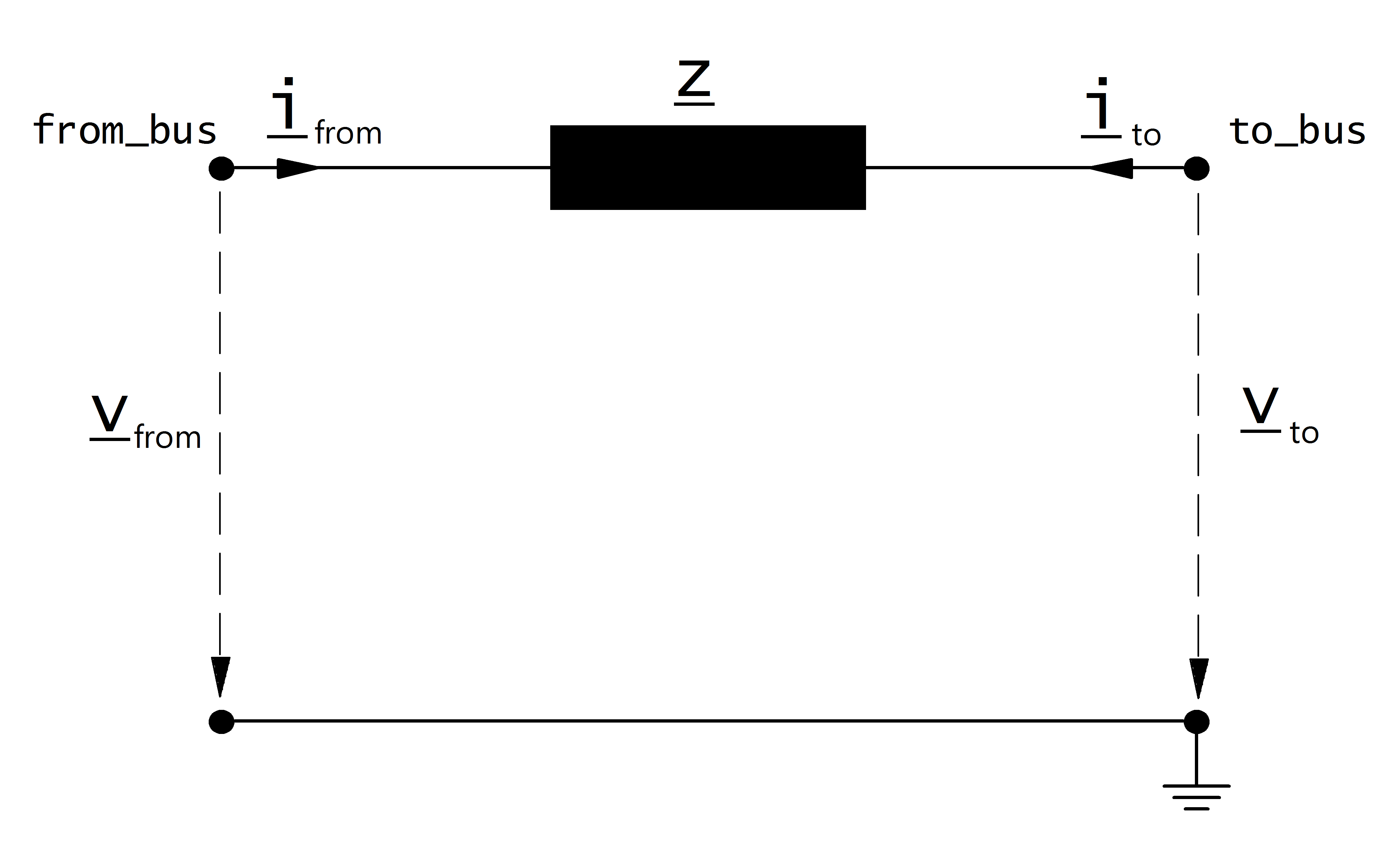# Impedance¶

## Create Function¶

pandapower.create_impedance(net, from_bus, to_bus, rft_pu, xft_pu, sn_mva, rtf_pu=None, xtf_pu=None, name=None, in_service=True, index=None)

Creates an per unit impedance element

INPUT:

net (pandapowerNet) - The pandapower network in which the element is created

from_bus (int) - starting bus of the impedance

to_bus (int) - ending bus of the impedance

r_pu (float) - real part of the impedance in per unit

x_pu (float) - imaginary part of the impedance in per unit

sn_mva (float) - rated power of the impedance in kVA

OUTPUT:

impedance id

## Input Parameters¶

net.impedance

 Parameter Datatype Value Range Explanation name string name of the impedance from_bus* integer index of bus where the impedance starts to_bus* integer index of bus where the impedance ends rft_pu* float $$>$$ 0 resistance of the impedance from ‘from’ to ‘to’ bus [p.u] xft_pu* float $$>$$ 0 reactance of the impedance from ‘from’ to ‘to’ bus [p.u] rtf_pu* float $$>$$ 0 resistance of the impedance from ‘to’ to ‘from’ bus [p.u] xtf_pu* float $$>$$ 0 reactance of the impedance from ‘to’ to ‘from’ bus [p.u] sn_mva* float $$>$$ 0 reference apparent power for the impedance per unit values [MVA] in_service* boolean True / False specifies if the imepdance is in service.

*necessary for executing a power flow calculation.

## Electric Model¶

The impedance is modelled as a longitudinal per unit impedance with $$\underline{z}_{ft} \neq \underline{z}_{tf}$$ :The per unit values given in the parameter table are assumed to be relative to the rated voltage of from and to bus as well as to the apparent power given in the table. The per unit values are therefore transformed into the network per unit system:

\begin{align*} \underline{z}_{ft} &= (rft\_pu + j \cdot xft\_pu) \cdot \frac{S_{N}}{sn\_mva} \\ \underline{z}_{tf} &= (rft\_pu + j \cdot xtf\_pu) \cdot \frac{S_{N}}{sn\_mva} \\ \end{align*}

where $$S_{N}$$ is the reference power of the per unit system (see Unit Systems and Conventions).

The asymetric impedance results in an asymetric nodal point admittance matrix:

\begin{bmatrix} Y_{00} & \dots & \dots & Y_{nn} \\ \vdots & \ddots & \underline{y}_{ft} & \vdots \\ \vdots & \underline{y}_{tf} & \ddots & \vdots \\ \underline{Y}_{n0} & \dots & \dots & \underline{y}_{nn}\\ \end{bmatrix}

## Result Parameters¶

net.res_impedance

 Parameter Datatype Explanation p_from_mw float active power flow into the impedance at “from” bus [MW] q_from_mvar float reactive power flow into the impedance at “from” bus [MVAr] p_to_mw float active power flow into the impedance at “to” bus [MW] q_to_mvar float reactive power flow into the impedance at “to” bus [MVAr] pl_mw float active power losses of the impedance [MW] ql_mvar float reactive power consumption of the impedance [MVar] i_from_ka float current at from bus [kA] i_to_ka float current at to bus [kA]
\begin{align*} i\_from\_ka &= i_{from}\\ i\_to\_ka &= i_{to}\\ p\_from\_mw &= Re(\underline{v}_{from} \cdot \underline{i}^*_{from}) \\ q\_from\_mvar &= Im(\underline{v}_{from} \cdot \underline{i}^*_{from}) \\ p\_to\_mw &= Re(\underline{v}_{to} \cdot \underline{i}^*_{to}) \\ q\_to\_mvar &= Im(\underline{v}_{to} \cdot \underline{i}^*_{to}) \\ pl\_mw &= p\_from\_mw + p\_to\_mw \\ ql\_mvar &= q\_from\_mvar + q\_to\_mvar \\ \end{align*}